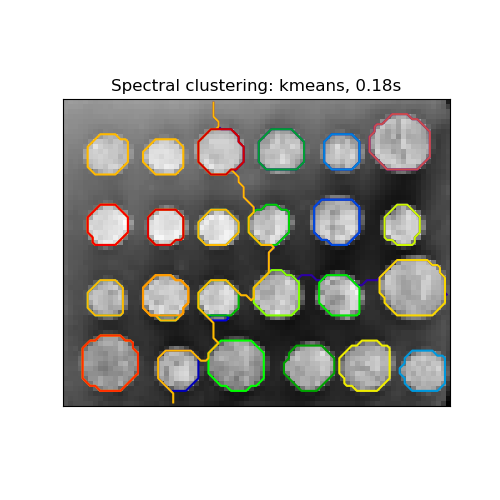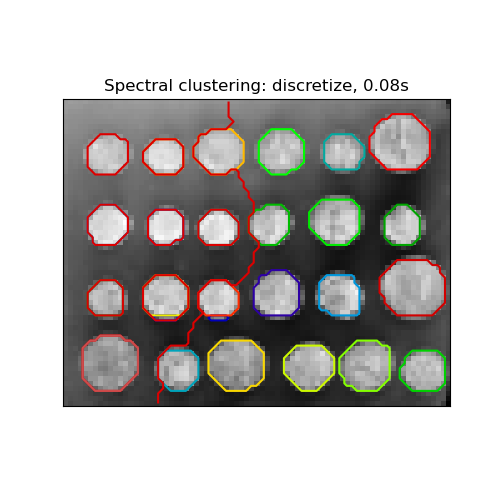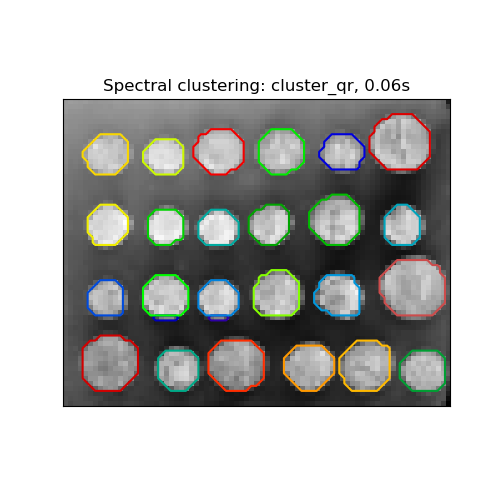# Segmenting the picture of greek coins in regions¶

This example uses Spectral clustering on a graph created from voxel-to-voxel difference on an image to break this image into multiple partly-homogeneous regions.

This procedure (spectral clustering on an image) is an efficient approximate solution for finding normalized graph cuts.

There are three options to assign labels:

• ‘kmeans’ spectral clustering clusters samples in the embedding space using a kmeans algorithm

• ‘discrete’ iteratively searches for the closest partition space to the embedding space of spectral clustering.

• ‘cluster_qr’ assigns labels using the QR factorization with pivoting that directly determines the partition in the embedding space.

# Author: Gael Varoquaux <gael.varoquaux@normalesup.org>
#         Brian Cheung
#         Andrew Knyazev <Andrew.Knyazev@ucdenver.edu>

import time

import numpy as np
from scipy.ndimage import gaussian_filter
import matplotlib.pyplot as plt
from skimage.data import coins
from skimage.transform import rescale

from sklearn.feature_extraction import image
from sklearn.cluster import spectral_clustering

# load the coins as a numpy array
orig_coins = coins()

# Resize it to 20% of the original size to speed up the processing
# Applying a Gaussian filter for smoothing prior to down-scaling
# reduces aliasing artifacts.
smoothened_coins = gaussian_filter(orig_coins, sigma=2)
rescaled_coins = rescale(smoothened_coins, 0.2, mode="reflect", anti_aliasing=False)

# Convert the image into a graph with the value of the gradient on the
# edges.
graph = image.img_to_graph(rescaled_coins)

# Take a decreasing function of the gradient: an exponential
# The smaller beta is, the more independent the segmentation is of the
# actual image. For beta=1, the segmentation is close to a voronoi
beta = 10
eps = 1e-6
graph.data = np.exp(-beta * graph.data / graph.data.std()) + eps

# The number of segmented regions to display needs to be chosen manually.
# The current version of 'spectral_clustering' does not support determining
# the number of good quality clusters automatically.
n_regions = 26


Compute and visualize the resulting regions

# Computing a few extra eigenvectors may speed up the eigen_solver.
# The spectral clustering quality may also benetif from requesting
# extra regions for segmentation.
n_regions_plus = 3

# Apply spectral clustering using the default eigen_solver='arpack'.
# Any implemented solver can be used: eigen_solver='arpack', 'lobpcg', or 'amg'.
# Choosing eigen_solver='amg' requires an extra package called 'pyamg'.
# The quality of segmentation and the speed of calculations is mostly determined
# by the choice of the solver and the value of the tolerance 'eigen_tol'.
# TODO: varying eigen_tol seems to have no effect for 'lobpcg' and 'amg' #21243.
for assign_labels in ("kmeans", "discretize", "cluster_qr"):
t0 = time.time()
labels = spectral_clustering(
graph,
n_clusters=(n_regions + n_regions_plus),
eigen_tol=1e-7,
assign_labels=assign_labels,
random_state=42,
)

t1 = time.time()
labels = labels.reshape(rescaled_coins.shape)
plt.figure(figsize=(5, 5))
plt.imshow(rescaled_coins, cmap=plt.cm.gray)

plt.xticks(())
plt.yticks(())
title = "Spectral clustering: %s, %.2fs" % (assign_labels, (t1 - t0))
print(title)
plt.title(title)
for l in range(n_regions):
colors = [plt.cm.nipy_spectral((l + 4) / float(n_regions + 4))]
plt.contour(labels == l, colors=colors)
# To view individual segments as appear comment in plt.pause(0.5)
plt.show()

# TODO: After #21194 is merged and #21243 is fixed, check which eigen_solver
# is the best and set eigen_solver='arpack', 'lobpcg', or 'amg' and eigen_tol
# explicitly in this example.

•••Spectral clustering: kmeans, 1.61s
Spectral clustering: discretize, 1.51s
Spectral clustering: cluster_qr, 1.51s


Total running time of the script: ( 0 minutes 4.985 seconds)

Gallery generated by Sphinx-Gallery